# Working with polygons

At the core of each SuperScreen simulation is an instance of the superscreen.Device class, which represents the superconducting structure to be modeled. A Device is composed of one or more Layers that each lie in a plane parallel to the $$x-y$$ plane (at position layer.z0) and have a specified penetration depth $$\Lambda$$. Each layer contains one or more superconducting films, and each film contains zero or more holes. Films and holes are represented by instances of the superscreen.Polygon class, which defines a 2D polygonal region.

:

# Automatically install superscreen from GitHub only if running in Google Colab
%pip install --quiet git+https://github.com/loganbvh/superscreen.git

:

%config InlineBackend.figure_formats = {"retina", "png"}
%matplotlib inline

import os

import numpy as np
import matplotlib.pyplot as plt

plt.rcParams["figure.figsize"] = (4, 4)
plt.rcParams["font.size"] = 10

import superscreen as sc

:

sc.version_table()

:

SoftwareVersion
SuperScreen0.10.2
Numpy1.25.2
Numba0.58.0
SciPy1.11.3
matplotlib3.8.0
IPython8.16.0
Python3.9.17 (main, Jul 27 2023, 09:05:49) [GCC 9.3.0]
OSposix [linux]
Number of CPUsPhysical: 1, Logical: 2
BLAS InfoOPENBLAS
Fri Sep 29 17:15:54 2023 UTC

A Polygon is defined by a collection of (x, y) coordinates specifying its vertices; the vertices are stored as an n x 2 numpy.ndarray called polygon.points.

:

# Define the initial geometry: a rectangular box specified as an np.ndarray
width, height = 10, 2
points = sc.geometry.box(width, height)
print(f"type(points) = {type(points)}, points.shape = {points.shape}")

type(points) = <class 'numpy.ndarray'>, points.shape = (100, 2)

:

# Create a Polygon representing a "horizontal bar", hbar
hbar = sc.Polygon(points=points)
hbar.polygon

:The object passed to sc.Polygon(points=...) can be of any of the following:

• An n x 2 array-like object, for example an np.ndarray or a list of (x, y) coordinates

• An existing sc.Polygon instance (in which case, the new object will be a copy of the existing one)

• An instance of LineString, LinearRing, or Polygon from the shapely package

:

(
sc.Polygon(points=hbar.points)
== sc.Polygon(points=hbar.polygon)
== sc.Polygon(points=hbar)
== hbar.copy()
== hbar
)

:

True


Every instance of sc.Polygon has a property, instance.polygon, which returns a corresponding shapely Polygon object. Among other things, this is usefuly for quickly visualizing polygons.

There are several methods for transforming the geometry of a single Polygon:

• polygon.translate(dx=0, dy=0)

• polygon.rotate(degrees, origin=(0, 0))

• polygon.scale(xfact=1, yfact=1, origin=(0, 0))

• polygon.buffer(distance, ...)

There are also three methods for combining multiple Polygon-like objects:

• polygon.union(*others): logical union of polygon with each object in the iterable others

• See also: sc.Polygon.from_union([...])

• polygon.intersection(*others): logical intersection of polygon with each object in the iterable others

• See also: sc.Polygon.from_intersection([...])

• polygon.difference(*others): logical difference of polygon with each object in the iterable others

• See also: sc.Polygon.from_difference([...])

Note that the elements of the iterable others can be of any type that can be passed in to sc.Polygon(points=...) (see above).

:

# Copy hbar and rotate the copy 90 degrees counterclockwise
vbar = hbar.rotate(90)
vbar.polygon

::

# Create a new Polygon that is the union of hbar and vbar: "+"
plus = hbar.union(vbar)
# # The above is equivalent to either of the following:
# plus = vbar.union(hbar)
# plus = sc.Polygon.from_union([hbar, vbar])
plus.polygon

::

# Rotate the "+" by 45 degrees to make an "X"
X = plus.rotate(45)
X.polygon

::

# Create a new polygon with all edges offset (eroded) by a distance of -0.5
thinX = X.buffer(-0.5)
thinX.polygon

::

# Create a new polygon with all edges offset (expanded) by a distance of 0.5
thickX = X.buffer(0.5)
thickX.polygon

::

polygons = [hbar, vbar, plus, X, thinX, thickX]
labels = ["hbar", "vbar", "plus", "X", "thinX", "thickX"]

fig, ax = plt.subplots(figsize=(6, 1))

for i, polygon in enumerate(polygons):
polygon.translate(dx=width * i).plot(ax=ax)

ax.set_xticks([width * i for i, _ in enumerate(labels)])
ax.set_xticklabels(labels)
_ = ax.set_yticks([])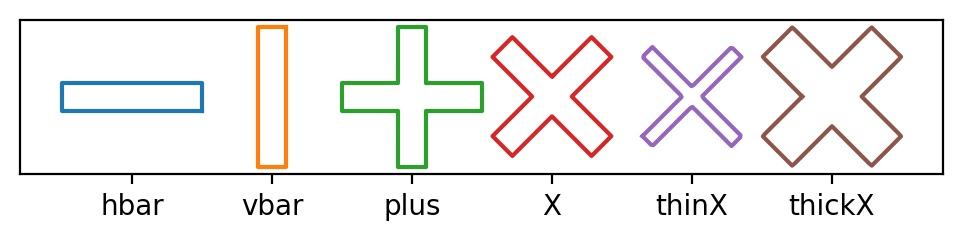:

X.union(plus).polygon

::

X.intersection(plus).polygon

:Using the methods demonstrated above, intricate geometries can be constructed from simple building blocks in just a few lines of code.

:

size = 10

hbar = sc.Polygon(points=sc.geometry.box(size / 3, size / 50))
plus = hbar.union(hbar.rotate(90))
star = plus.union(plus.rotate(45))

star_dx = 1.2 * size * np.sqrt(2) / 2

snowflake = (
sc.Polygon(points=sc.geometry.box(size, size))
.rotate(45)
.union(
*(star.translate(dx=star_dx).rotate(degrees) for degrees in [0, 90, 180, 270])
)
)
snowflake = snowflake.union(snowflake.rotate(45))

:

ax = snowflake.plot()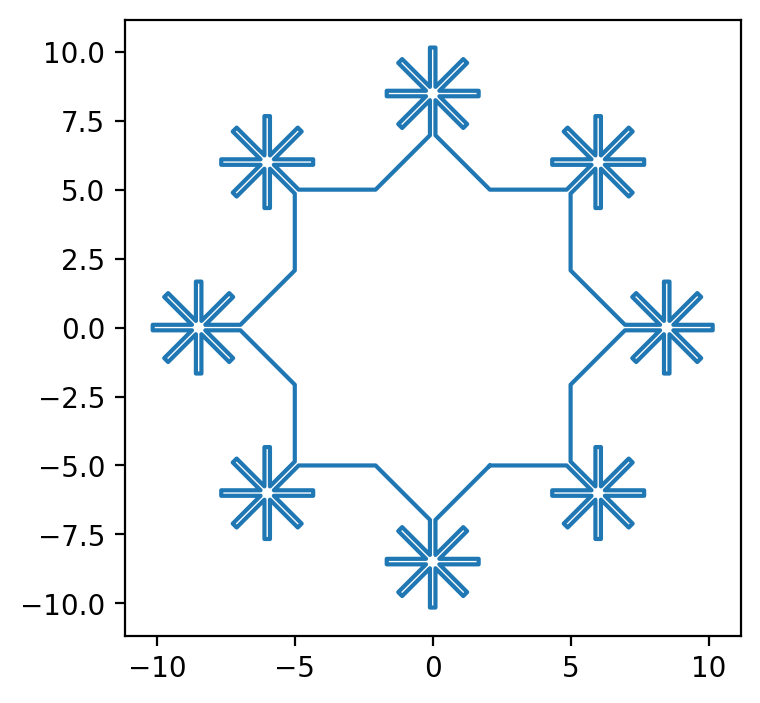:

print(f"Polygon area: snowflake.area = {snowflake.area:.3f}")
print(f"Polygon width and height: snowflake.extents = {snowflake.extents}")

Polygon area: snowflake.area = 136.622
Polygon width and height: snowflake.extents = (20.303896081810475, 20.303896081810475)


In SuperScreen, all polygons must be simply-connected, i.e. have no holes.

:

circle = sc.Polygon(points=sc.geometry.circle(10))
hole = circle.scale(0.5, 0.5)
try:
ring = circle.difference(hole)
except ValueError as e:
print(f"ValueError('{e}')")

ValueError('Expected a simply-connected polygon.')


However, SuperScreen does allow you to model multiply-connected films. A film with $$h$$ holes in it is modeled as $$h + 1$$ separate sc.Polygon objects:

:

h = 9
circle = sc.Polygon(points=sc.geometry.circle(5))
holes = [
sc.Polygon(points=sc.geometry.box(2, 1)).translate(3.5).rotate(theta)
for theta in np.linspace(0, 360, h, endpoint=False)
]

ax = circle.plot(lw=3)
for hole in holes:
hole.plot(ax=ax, lw=3)
# Boolean array indicating whether each point in hole.points
# lies within the circle
points_in_circle = circle.contains_points(hole.points)
assert isinstance(points_in_circle, np.ndarray)
assert points_in_circle.shape == hole.points.shape
assert points_in_circle.dtype == np.dtype(bool)
assert points_in_circle.all()

# Alternatively, use shapely to check whehther the hole's polygon
# lies within the circle's polygon
assert circle.polygon.contains(hole.polygon)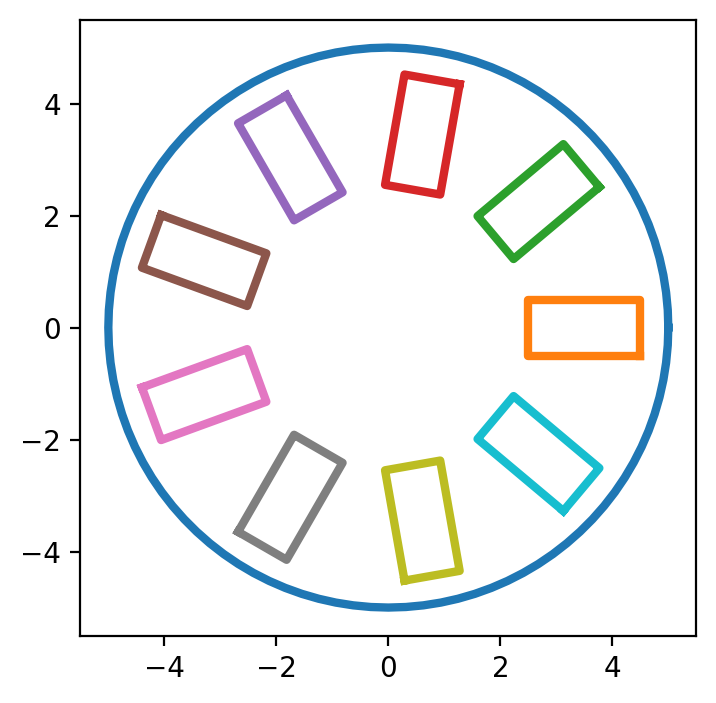## Meshing Polygons

Individual polygons can be meshed using the Polygon.make_mesh() method.

:

setups = [
dict(min_points=500, smooth=0),
dict(max_edge_length=0.5, smooth=100),
]

fig, axes = plt.subplots(1, len(setups), figsize=(2 * (len(setups) + 0.5), 2))

for ax, options in zip(axes, setups):
# Generate a mesh with the specified options
mesh = X.make_mesh(**options)

# Plot the mesh
ax.set_aspect("equal")
title = [f"{key}={value!r}" for key, value in options.items()]
title.append(f"Actual points = {len(mesh.sites)}")
ax.triplot(mesh.sites[:, 0], mesh.sites[:, 1], mesh.elements, linewidth=0.75)
ax.set_title("\n".join(title))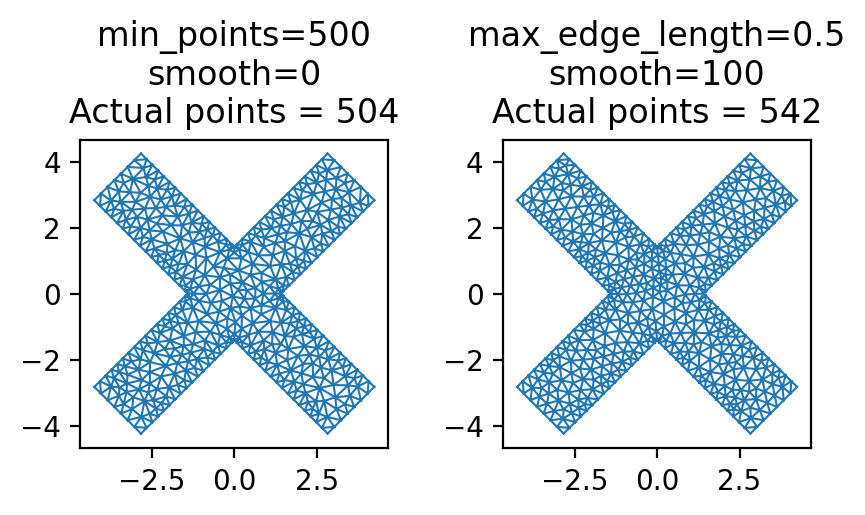This can come in handy if, for example, you want to use a Polygon to define an applied magnetic field rather than include it in a Device to solve.

Here we calculate the vector magnetic field from a magnetic “X” lying in the $$x-y$$ plane with uniform out-of-plane magnetization. We’ll assume that the dimensions of the “X” are in microns and that its magnetization (area density of magnetic moments) is $$\vec{m}=1\,\mu_\mathrm{B}/\mathrm{nm}^2\,\hat{z}$$, where $$\mu_\mathrm{B}$$ is the Bohr magneton.

:

# Generate the mesh
mesh = X.make_mesh(min_points=1000)
x, y = mesh.sites[:, 0], mesh.sites[:, 1]

# (x, y, z) position of each vertex
vertex_positions = np.stack([x, y, np.zeros_like(x)], axis=1)

# Calculate the effective area of each vertex in the mesh
vertex_areas = mesh.vertex_areas * sc.ureg("um ** 2")

# Define the magnetic moment of each mesh vertex
z_hat = np.array([0, 0, 1])  # unit vector in the z direction
magnetization = sc.ureg("1 mu_B / nm ** 2")
vertex_moments = z_hat * magnetization * vertex_areas[:, np.newaxis]
# Convert to units of Bohr magnetons
vertex_moments = vertex_moments.to("mu_B").magnitude


The function superscreen.sources.DipoleField returns a superscreen.Parameter that calulates the magnetic field (in Tesla) from a disribution of magnetic dipoles.

:

field_param = sc.sources.DipoleField(
dipole_positions=vertex_positions,
dipole_moments=vertex_moments,
)


Evaluate the magnetic field from the “X”:

:

# Coordinates at which to evaluate the magnetic field (in microns)
N = 101
eval_xs = eval_ys = np.linspace(-5, 5, N)
eval_z = 0.5
xgrid, ygrid, zgrid = np.meshgrid(eval_xs, eval_ys, eval_z)
xgrid = np.squeeze(xgrid)
ygrid = np.squeeze(ygrid)
zgrid = np.squeeze(zgrid)

# field_param returns shape (N * N, 3), where the last axis is field component
# and the units are Tesla
field = field_param(xgrid.ravel(), ygrid.ravel(), zgrid.ravel()) * sc.ureg("tesla")
# Reshape to (N, N, 3), where the last axis is field component,
# and convert to microTesla
field = field.reshape((N, N, 3)).to("microtesla").magnitude


Plot the results:

:

fig, axes = plt.subplots(
1, 3, figsize=(6, 2), sharex=True, sharey=True, constrained_layout=True
)

vmax = max(abs(field.min()), abs(field.max()))
vmin = -vmax
kwargs = dict(vmin=vmin, vmax=vmax, shading="auto", cmap="coolwarm")

for i, (ax, label) in enumerate(zip(axes, "xyz")):
ax.set_aspect("equal")
im = ax.pcolormesh(xgrid, ygrid, field[..., i], **kwargs)
ax.set_title(f"$\\mu_0H_{{{label}}}(z={{{eval_z}}}\\,\\mu\\mathrm{{m}})$")
cbar = fig.colorbar(im, ax=axes)
cbar.set_label("$\\mu_0H$ [$\\mu$T]")
_ = axes.set_xlabel("$x$ [$\\mu$m]")
_ = axes.set_ylabel("$y$ [$\\mu$m]")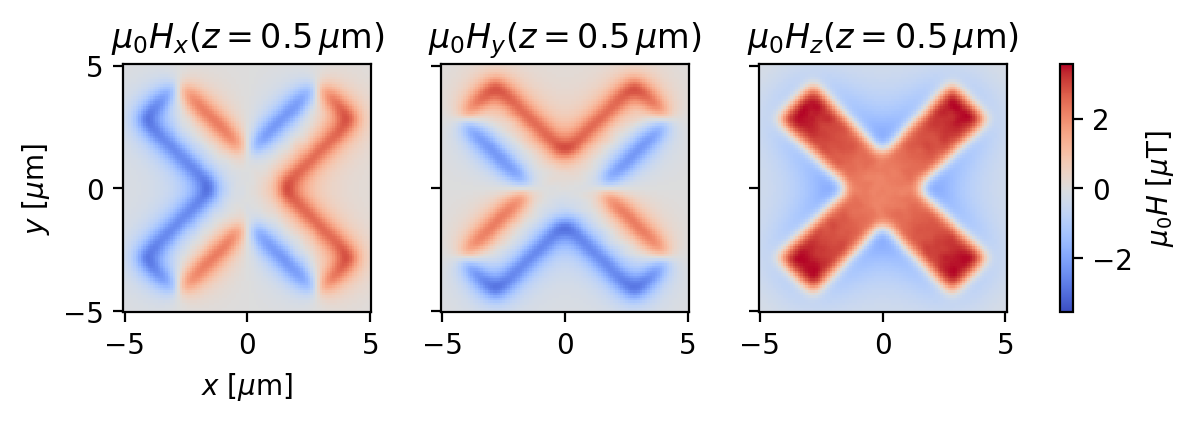[ ]: